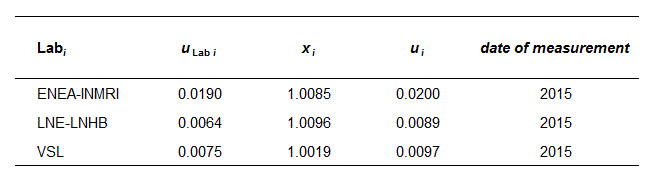Comparison
Comparison close
Results

BIPM.RI(I)-K9 and EURAMET.RI(I)-K9

MEASURAND : Absorbed dose to water

Degrees of equivalence, Di  and its expanded uncertainty Ui (k = 2), both expressed in mGy/Gy.

100 kV
Di
Participating institutes

BIPM.RI(I)-K9 and EURAMET.RI(I)-K9

MEASURAND  Absorbed dose to water

Degrees of equivalence, Di and expanded uncertainty Ui (k = 2), expressed in mGy/Gy

135 kV
Di
Participating institutes

BIPM.RI(I)-K9 and EURAMET.RI(I)-K9

MEASURAND   Absorbed dose to water

Degrees of equivalence, Di and its expanded uncertainty Ui (k = 2), both expressed in mGy/Gy.

180 kV
Di
Participating institutes

BIPM.RI(I)-K9 and EURAMET.RI(I)-K9

MEASURAND   Absorbed dose to water

Degrees of equivalence, Di and its expanded uncertainty Ui  (k = 2), both expressed in mGy/Gy.

250 kV
Di
Participating institutes
Comparison
Comparison close
Results

BIPM.RI(I)-K9 and EURAMET.RI(I)-K9

MEASURAND : Absorbed dose to water

Degrees of equivalence, Di  and its expanded uncertainty Ui (k = 2), both expressed in mGy/Gy.

LABi Di Uneg,i Upos,i
mGy/Gy
mGy/Gy
mGy/Gy
PTB -6.7 21.4
LNE-LNHB -22.1 23.8
VSL -5.4 20.7
Participating institutes
BIPM.RI(I)-K9 100 kV
EURAMET.RI(I)-K9 100 kV

BIPM.RI(I)-K9 and EURAMET.RI(I)-K9

MEASURAND : Absorbed dose to water

Degrees of equivalence, Di and expanded uncertainty Ui (k = 2), expressed in mGy/Gy

LABi Di Uneg,i Upos,i
mGy/Gy
mGy/Gy
mGy/Gy
PTB -11.1 20.2
LNE-LNHB -1.1 18
VSL 9.9 19.6
Participating institutes
BIPM.RI(I)-K9 135 kV
EURAMET.RI(I)-K9 135 kV

BIPM.RI(I)-K9 and EURAMET.RI(I)-K9

MEASURAND   Absorbed dose to water

Degrees of equivalence, Di and expanded uncertainty Ui (k = 2), expressed in mGy/Gy

LABi Di Uneg,i Upos,i
mGy/Gy
mGy/Gy
mGy/Gy
PTB -9.4 17.8
LNE-LNHB -15.5 21
ENEA-INMRI -7.7 40
VSL -1.4 19
Participating institutes
BIPM.RI(I)-K9 180 kV
EURAMET.RI(I)-K9 180 kV

BIPM.RI(I)-K9 and EURAMET.RI(I)-K9

MEASURAND   Absorbed dose to water

Degrees of equivalence, Di and its expanded uncertainty Ui (k = 2), both expressed in mGy/Gy.

LABi Di Uneg,i Upos,i
mGy/Gy
mGy/Gy
mGy/Gy
PTB -5.3 17.8
LNE-LNHB 7.4 18
ENEA-INMRI 8.5 40
VSL 1.9 19.5
Participating institutes
BIPM.RI(I)-K9 250 kV
EURAMET.RI(I)-K9 250 kV
Comparison
Comparison close
BIPM comparison
 Metrology area, Sub-field Ionizing Radiation, Section I (x and gamma rays, electrons) Description Measurement of absorbed dose to water for medium energy X-rays Time of measurements 2016 - Status Continuous, approved for equivalence
 Measurand Absorbed dose to water relative to the BIPM evaluation Parameters Radiation type: X-rays Radiation quality: 100 kV to 250 kV Transfer device Ionization chambers
 Comparison type Key Comparison Consultative Committee CCRI (Consultative Committee for Ionizing Radiation) Conducted by BIPM (Bureau International des Poids et Mesures) Comments The Final Report for each bi-lateral comparsion is listed in the tab "Participants" First results published on 17 April 2018. Last update made on 27 March 2020. Pilot institute BIPM Bureau International des Poids et Mesures BIPM - International Organization Contact person D. T. Burns +33 (0) 1 45 07 70 80
First Name Last Name
wwww@ww.www +356719836 Institute 1 Institute 1 Khmelnitskiy
Pilot laboratory
BIPM

Bureau International des Poids et Mesures, BIPM - International Organization, N/A

 LNE-LNHB Commissariat à l'énergie atomique / Laboratoire National Henri Becquerel, France, EURAMET 2018 -
 PTB Physikalisch-Technische Bundesanstalt, Germany, EURAMET 2016
Comparison
Comparison close
Results

BIPM.RI(I)-K9 and EURAMET.RI(I)-K9

MEASURAND : Absorbed dose to water

For a comparison with laboratory i, the key comparison reference value is the BIPM determination xR,i and its standard uncertainty uR,i.

The degree of equivalence of laboratory i with respect to xR,i is given by a pair of terms: Di and Ui = 2 ui, its expanded uncertainty (k = 2), both expressed in mGy/Gy.
In evaluating ui for BIPM comparisons, account is taken of correlation between uLab i and uR,i (see the relevant Final Report). For regional comparisons, additional linking uncertainties are included.

When required, the degree of equivalence between two laboratories i and j can be evaluated by a pair of terms: Dij = Di - Dj and Uij = 2uij, its expanded uncertainty (k = 2), both expressed in mGy/Gy. In evaluating uij, account should be taken of correlation between ui and uj. For regional comparisons involving secondary standards, this includes correlation related to traceability.

BIPM.RI(I)-K9 and EURAMET.RI(I)-K9

MEASURAND   Absorbed dose to water

For a comparison with laboratory i, the key comparison reference value is the BIPM determination xR,i and its standard uncertainty uR,i.

The degree of equivalence of laboratory i with respect to xR,i is given by a pair of terms: Di and Ui = 2 ui, its expanded uncertainty (k = 2), both expressed in mGy/Gy.
In evaluating ui for BIPM comparisons, account is taken of correlation between uLab i and uR,i  (see the relevant Final Report). For regional comparisons, additional linking uncertainties are included.

When required, the degree of equivalence between two laboratories i and j can be evaluated by a pair of terms: Dij = Di - Dj and Uij = 2uij, its expanded uncertainty (k = 2), both expressed in mGy/Gy. In evaluating uij, account should be taken of correlation between ui and uj. For regional comparisons involving secondary standards, this includes correlation related to traceability.

BIPM.RI(I)-K9 and EURAMET.RI(I)-K9

MEASURAND   Absorbed dose to water

For a comparison with laboratory i, the key comparison reference value is the BIPM determination xR,i and its standard uncertainty uR,i.

The degree of equivalence of laboratory i with respect to xR,i is given by a pair of terms: Di and Ui = 2 ui, its expanded uncertainty (k = 2), both expressed in mGy/Gy.
In evaluating ui for BIPM comparisons, account is taken of correlation between uLab i and uR,i (see the relevant Final Report). For regional comparisons, additional linking uncertainties are included.

When required, the degree of equivalence between two laboratories i and j can be evaluated by a pair of terms: Dij = Di - Dj and Uij = 2uij, its expanded uncertainty (k = 2), both expressed in mGy/Gy. In evaluating uij, account should be taken of correlation between ui and uj. For regional comparisons involving secondary standards, this includes correlation related to traceability.

BIPM.RI(I)-K9 and EURAMET.RI(I)-K9

MEASURAND   Absorbed dose to water

For a comparison with laboratory i, the key comparison reference value is the BIPM determination xR,i and its standard uncertainty uR,i.

The degree of equivalence of laboratory i with respect to xR,i is given by a pair of terms: Di and Ui = 2 ui, its expanded uncertainty (k = 2), both expressed in mGy/Gy.
In evaluating ui for BIPM comparisons, account is taken of correlation between uLab i and uR,i (see the relevant Final Report). For regional comparisons, additional linking uncertainties are included.

When required, the degree of equivalence between two laboratories i and j can be evaluated by a pair of terms: Dij = Di - Dj and Uij = 2uij, its expanded uncertainty (k = 2), both expressed in mGy/Gy. In evaluating uij, account should be taken of correlation between ui and uj. For regional comparisons involving secondary standards, this includes correlation related to traceability.

Comparison
Comparison close
Results

BIPM.RI(I)-K9 and EURAMET.RI(I)-K9

MEASURAND : Absorbed dose to water

BIPM.RI(I)-K9

uLabi  relative standard uncertainty of absorbed dose to water determined by Lab i

xi   absorbed dose to water determined by Labi at the BIPM, relative to the reference value determined by the BIPM standard

ui   combined standard uncertainty of xi taking correlation into account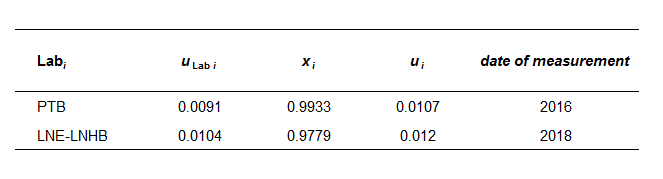EURAMET.RI(I)-K9

uLab i : relative standard uncertainty of calibration coefficients determined by Lab i

xi : absorbed dose to water determined by Lab i at the BIPM, relative to the reference value determined by the BIPM standard, derived from the calibration coefficients through the results of the linking laboratory (PTB) as detailed in the EURAMET.RI(I)-K9 Final Report.

ui : combined standard uncertainty of xi taking correlation into account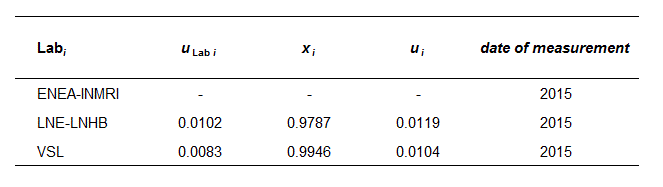BIPM.RI(I)-K9 and EURAMET.RI(I)-K9

MEASURAND : Absorbed dose to water

BIPM.RI(I)-K9

uLab i : relative standard uncertainty of absorbed dose to water determined by Lab i

xi : absorbed dose to water determined by Lab i at the BIPM, relative to the reference value determined by the BIPM standard

ui : combined standard uncertainty of xi taking correlation into account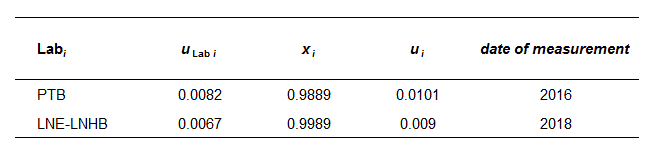EURAMET.RI(I)-K9

uLab i : relative standard uncertainty of calibration coefficients determined by Lab i

xi : absorbed dose to water determined by Lab i at the BIPM, relative to the reference value determined by the BIPM standard, derived from the calibration coefficients through the results of the linking laboratory (PTB) as detailed in the EURAMET.RI(I)-K9 Final Report.

ui : combined standard uncertainty of xi taking correlation into account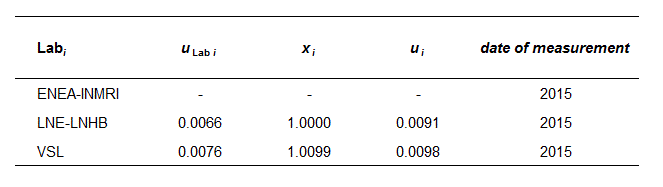BIPM.RI(I)-K9 and EURAMET.RI(I)-K9

MEASURAND   Absorbed dose to water

BIPM.RI(I)-K9

uLab i : relative standard uncertainty of absorbed dose to water determined by Lab i

xi : absorbed dose to water determined by Lab i at the BIPM, relative to the reference value determined by the BIPM standard

ui : combined standard uncertainty of xi taking correlation into account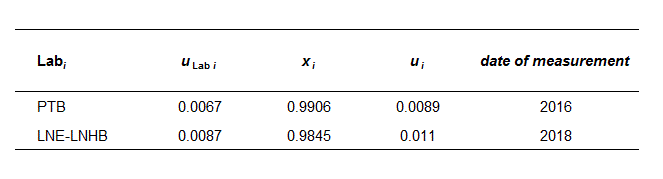EURAMET.RI(I)-K9

uLab i : relative standard uncertainty of calibration coefficients determined by Lab i

xi : absorbed dose to water determined by Lab i at the BIPM, relative to the reference value determined by the BIPM standard, derived from the calibration coefficients through the results of the linking laboratory (PTB) as detailed in the EURAMET.RI(I)-K9 Final Report.

ui : combined standard uncertainty of xi taking correlation into account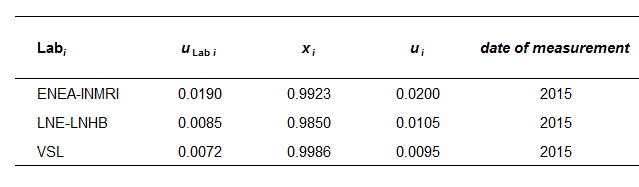BIPM.RI(I)-K9 and EURAMET.RI(I)-K9

MEASURAND  Absorbed dose to water

BIPM.RI(I)-K9

uLab i : relative standard uncertainty of absorbed dose to water determined by Lab i

xi : absorbed dose to water determined by Lab i at the BIPM, relative to the reference value determined by the BIPM standard

ui : combined standard uncertainty of xi taking correlation into account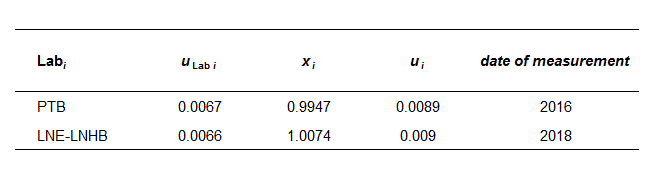EURAMET.RI(I)-K9

uLab i : relative standard uncertainty of calibration coefficients determined by Lab i

xi : absorbed dose to water determined by Lab i at the BIPM, relative to the reference value determined by the BIPM standard, derived from the calibration coefficients through the results of the linking laboratory (PTB) as detailed in the EURAMET.RI(I)-K9 Final Report.

ui : combined standard uncertainty of xi taking correlation into account